# Counters

## Digital Circuits

• #### Question 1

 Don’t just sit there! Build something!!

Learning to analyze digital circuits requires much study and practice. Typically, students practice by working through lots of sample problems and checking their answers against those provided by the textbook or the instructor. While this is good, there is a much better way.

You will learn much more by actually building and analyzing real circuits, letting your test equipment provide the “answers” instead of a book or another person. For successful circuit-building exercises, follow these steps:

1. Draw the schematic diagram for the digital circuit to be analyzed.
2. Carefully build this circuit on a breadboard or other convenient medium.
3. Check the accuracy of the circuit’s construction, following each wire to each connection point, and verifying these elements one-by-one on the diagram.
4. Analyze the circuit, determining all output logic states for given input conditions.
5. Carefully measure those logic states, to verify the accuracy of your analysis.
6. If there are any errors, carefully check your circuit’s construction against the diagram, then carefully re-analyze the circuit and re-measure.

Always be sure that the power supply voltage levels are within specification for the logic circuits you plan to use. If TTL, the power supply must be a 5-volt regulated supply, adjusted to a value as close to 5.0 volts DC as possible.

One way you can save time and reduce the possibility of error is to begin with a very simple circuit and incrementally add components to increase its complexity after each analysis, rather than building a whole new circuit for each practice problem. Another time-saving technique is to re-use the same components in a variety of different circuit configurations. This way, you won’t have to measure any component’s value more than once.

• #### Question 2

Count from zero to fifteen, in binary, keeping the bits lined up in vertical columns like this:

0000

0001

0010

. . .

Now, reading from top to bottom, notice the alternating patterns of 0’s and 1’s in each place (i.e. one’s place, two’s place, four’s place, eight’s place) of the four-bit binary numbers. Note how the least significant bit alternates more rapidly than the most significant bit. Draw a timing diagram showing the respective bits as waveforms, alternating between “low” and “high” states, and comment on the frequency of each of the bits.

• #### Question 3

Shown here is a simple two-bit binary counter circuit:The Q output of the first flip-flop constitutes the least significant bit (LSB), while the second flip-flop’s Q output constitutes the most significant bit (MSB).

Based on a timing diagram analysis of this circuit, determine whether it counts in an up sequence (00, 01, 10, 11) or a down sequence (00, 11, 10, 01). Then, determine what would have to be altered to make it count in the other direction.

• #### Question 4

Counter circuits built by cascading the output of one flip-flop to the clock input of the next flip-flop are generally referred to as ripple counters. Explain why this is so. What happens in such a circuit that earns it the label of “ripple”? Is this effect potentially troublesome in circuit operation, or is it something of little or no consequence?

• #### Question 5

A style of counter circuit that completely circumvents the “ripple” effect is called the synchronous counter:Complete a timing diagram for this circuit, and explain why this design of counter does not exhibit “ripple” on its output lines: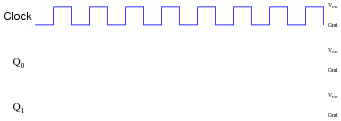Challenge question: to really understand this type of counter circuit well, include propagation delays in your timing diagram.

• #### Question 6

A student just learned how a two-bit synchronous binary counter works, and he is excited about building his own. He does so, and the circuit works perfectly.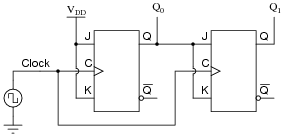After that success, student tries to expand on their success by adding more flip-flops, following the same pattern as the two original flip-flops:Unfortunately, this circuit didn’t work. The sequence it generates is not a binary count. Determine what the counting sequence of this circuit is, and then try to figure out what modifications would be required to make it count in a proper binary sequence.

• #### Question 7

Complete a timing diagram for this synchronous counter circuit, and identify the direction of its binary count: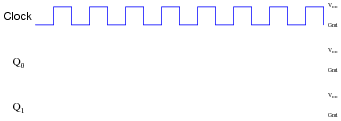• #### Question 8

Synchronous counter circuits tend to confuse students. The circuit shown here is the design that most students think ought to work, but actually doesn’t: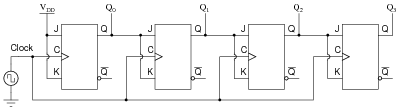Shown here is an up/down synchronous counter design that does work:Explain why this circuit is able to function properly (counting in either direction), while the first circuit is not able to count properly at all. What do those “extra” gates do to make the counter circuit function as it should. Hint: to more easily compare the up/down counter to the faulty up counter initially shown, connect the Up/[Down] control line high, and then disregard any lines and gates that become disabled as a result.

• #### Question 9

The following circuit is a two-bit synchronous binary up/down counter:Explain what would happen if the upper AND gate’s output were to become “stuck” in the high state regardless of its input conditions. What effect would this kind of failure have on the counter’s operation?

• #### Question 10

Supposed we used J-K flip-flops with asynchronous inputs (Preset and Clear) to build a counter:With the asynchronous lines paralleled as such, what are we able to make the counter do now that we weren’t before we had asynchronous inputs available to us?

• #### Question 11

The part number 74HCT163 integrated circuit is a high-speed CMOS, four-bit, synchronous binary counter. It is a pre-packaged unit, will all the necessary flip-flops and selection logic enclosed to make your design work easier than if you had to build a counter circuit from individual flip-flops. Its block diagram looks something like this (power supply terminals omitted, for simplicity):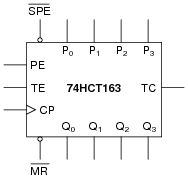Research the function of this integrated circuit, from manufacturers’ datasheets, and explain the function of each input and output terminal.

• #### Question 12

Determine the output pulses for this counter circuit, known as a Johnson counter, assuming that all Q outputs begin in the low state: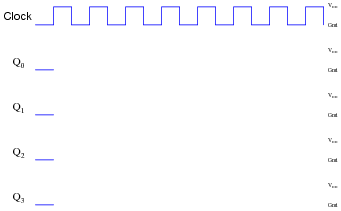• #### Question 13

The following circuit is known as a Johnson counter:Describe the output of this circuit, as measured from the Q output of the far right flip-flop, assuming that all flip-flops power up in the reset condition.

Also, explain what this modified version of the above Johnson counter circuit will do, in each of the five selector switch positions:• #### Question 14

Suppose we had two four-bit synchronous up/down counter circuits, which we wished to cascade to make one eight-bit counter. Draw the necessary connecting wires (and any extra gates) between the two four-bit counters to make this possible:After deciding how to cascade these counters, imagine that you are in charge of building and packaging four-bit counter circuits. The customers who buy your counters might wish to cascade them as you did here, but they won’t have the ability to “go inside” the packaging as you did to connect to any of the lines between the various flip-flops. This means you will have to provide any necessary cascading lines as inputs and outputs on your pre-packaged counters. Think carefully about how you would choose to build and package your four-bit “cascadable” counters, and then draw a schematic diagram.

• #### Question 15

Here is an eight-bit counter comprised of two four-bit 74HCT163 synchronous binary counters cascaded together: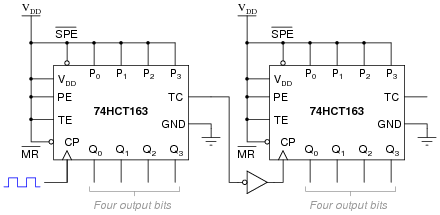Explain how this counter circuit works, and also determine which output bit is the LSB and which is the MSB.

Now, examine this eight-bit counter comprised of the same two ICs: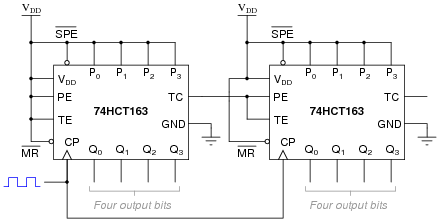Explain how this counter circuit works, and how its operation differs from the previous eight-bit counter circuit.

• #### Question 16

A student wishes to cascade multiple four-bit synchronous counters together. His first effort looks like this, and it works well as an eight-bit counter: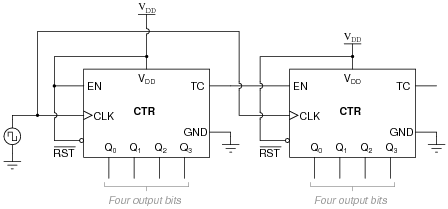Encouraged by this success, the student decides to add another four-bit counter to the end to make a twelve-bit counter circuit: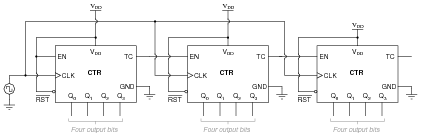Unfortunately, this arrangement does not work so well. It seems to work good for the first 241 counts (from 000000000000 to 000011110000), but then the last four bits begin to cycle as quickly as the first four bits, while the middle four bits remain in the 1111 state for 15 additional clock pulses. Something is definitely very wrong here!

Determine what the problem is, and suggest a remedy for it. Hint: this situation is very similar to connecting more than two J-K flip-flops together to form a synchronous counter circuit.

• #### Question 17

Some integrated circuit counters come equipped with multiple enable inputs. A good example of this is the 74HCT163: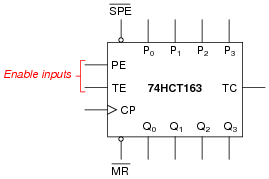In this case, as in others, the two enable inputs are not identical. Although both must be active for the counter to count, one of the enable inputs does something extra that the other one does not. This additional function is often referred to as a look-ahead carry, provided to simplify cascading of counters.

Explain what “look-ahead carry” means in the context of digital counter circuits, and why it is a useful feature.

• #### Question 18

Determine the modulus (MOD) of a four-bit binary counter. Determine the modulus of two four-bit binary counters cascaded to make an eight-bit binary counter.

• #### Question 19

Consider the following four-bit binary counter integrated circuit (IC). When clocked by the square wave signal generator, it counts from 0000 to 1111 in sixteen steps and then “recycles” back to 0000 again in a single step: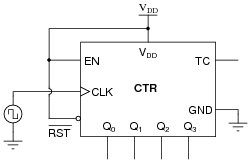There are many applications, though, where we do not wish the counter circuit to count all the way up to full count (1111), but rather recycle at some lesser terminal count value. Take for instance the application of BCD counting: from 0000 to 1001 and back again. Here is one way to truncate the counting sequence of a binary counter so that it becomes a BCD counter:Explain how the NAND gate forces this counter to recycle after an output of 1001 instead of counting all the way up to 1111. (Hint: the reset function of this IC is assumed to be asynchronous, meaning the counter output resets to 0000 immediately when the [RST] terminal goes low.)

Also, show how you would modify this circuit to do the same count sequence (BCD) assuming the IC has a synchronous reset function, meaning the counter resets to 0000 if [RST] is low and the clock input sees a pulse.

• #### Question 20

Suppose you had an astable multivibrator circuit that output a very precise 1 Hz square-wave signal, but you had an application which requires a pulse once every minute rather than once every second. Knowing that there are 60 seconds in a minute, can you think of a way to use digital counters to act as a “frequency divider” so that every 60 multivibrator pulses equates to 1 output pulse?

You don’t have a divide-by-60 counter available, but you do have several divide-by-10 (“decade”) counters at your disposal. Engineer a solution using these counter units: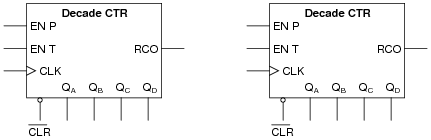Note: assume these counter ICs have asynchronous resets.

• #### Question 21

When counters are used as frequency dividers, they are often drawn as simple boxes with one input and one output each, like this: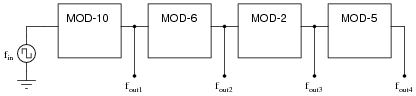Calculate the four output frequencies (fout1 through fout4) given an input frequency of 1.5 kHz:

fout1 =
fout2 =
fout3 =
fout4 =
• #### Question 22

A student builds a four-bit asynchronous counter circuit using CMOS J-K flip-flops. It seems to work . . . most of the time. Every once in a while, the count suddenly and mysteriously “jumps” out of sequence, to a value that is completely wrong. Even stranger than this is the fact that it seems to happen every time the student waves their hand next to the circuit.

What do you suspect the problem to be?

• #### Question 23

Identify a single fault that would allow this synchronous counter circuit to count up on demand, but not down: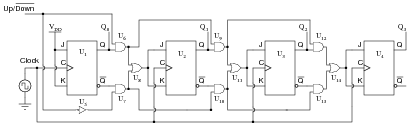Explain why your proposed fault would cause the problem.

• #### Question 24

A student builds a four-bit asynchronous up counter out of individual J-K flip-flops, but is dissatisfied with its performance: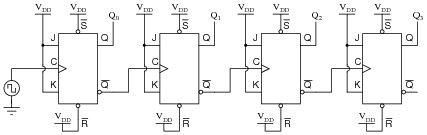Although the counting sequence is proper, the circuit usually does not begin counting from 0000 at power-up. The fact that the circuit counts correctly suggests that there is nothing failed or mis-wired, so what could possibly be wrong?

• #### Question 25

The following RC circuit constitutes an automatic reset network for the counter. At power-up, it resets the counter to 0000, then allows it to count normally:Predict how the operation of this automatic reset circuit will be affected as a result of the following faults. Consider each fault independently (i.e. one at a time, no multiple faults):

Resistor R1 fails open:
Resistor R2 fails open:
Resistor R3 fails open:
Capacitor C1 fails shorted:

For each of these conditions, explain why the resulting effects will occur.

• #### Question 26

A student is trying to get a 74HC192 up/down counter to function. However, it is simply not cooperating:Determine what the student is doing wrong with this 74HC192, and then correct the schematic diagram.

• #### Question 27

This Johnson counter circuit is special. It outputs three square-wave signals, shifted 120o from one another: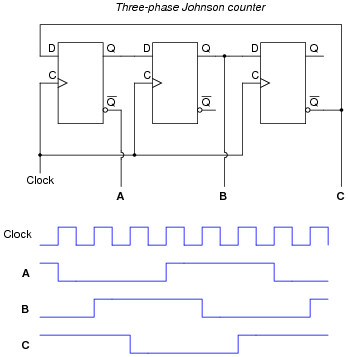Suppose the middle flip-flop’s Q output fails in the “high” state. Plot the new output waveforms for signals A, B, and C. Assume all Q outputs begin in the “low” state (except for the middle flip-flop, of course):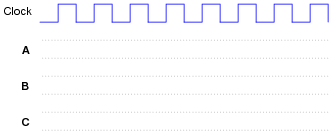• #### Question 28

A technician is trying to build a timer project using a set of cascaded counters, each one connected to its own 7-segment decoder and display: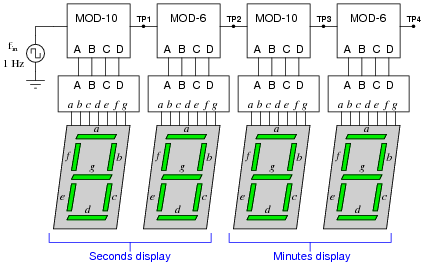The technician was trying to troubleshoot this circuit, but left without finishing the job. You were sent to finish the work, having only been told that the timer circuit “has some sort of problem.” Your first step is to start the 1 Hz clock and watch the timing sequence, and after a few minutes of time you fail to notice anything out of the ordinary.

Now, you could sit there for a whole hour and watch the count sequence, but that might take a long time before anything unusual appears for you to see. Devise a test procedure that will allow you to pinpoint problems at a much faster rate.

• #### Question 29

Explain the difference between a synchronous counter and an asynchronous counter circuit.

• #### Question 30

Draw the schematic diagram for a four-bit binary “up” counter circuit, using J-K flip-flops.

• #### Question 31

Complete a timing diagram for this circuit, and determine its direction of count, and also whether it is a synchronous counter or an asynchronous counter: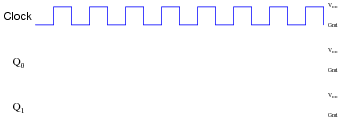• #### Question 32

Complete a timing diagram for this circuit, and determine its direction of count, and also whether it is a synchronous counter or an asynchronous counter: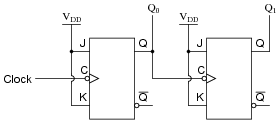• #### Question 33

Complete a timing diagram for this circuit, and determine its direction of count, and also whether it is a synchronous counter or an asynchronous counter: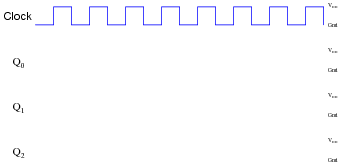• #### Question 34

When counters are used as frequency dividers, they are often drawn as simple boxes with one input and one output each, like this:Calculate the four output frequencies (fout1 through fout4) given an input frequency of 25 kHz:

fout1 =
fout2 =
fout3 =
fout4 =